1. /
2. CBSE
3. /
4. Class 08
5. /
6. Mathematics
7. /
8. NCERT Solutions for Class...

# NCERT Solutions for Class 8 Maths Exercise 11.4### myCBSEguide App

Download the app to get CBSE Sample Papers 2023-24, NCERT Solutions (Revised), Most Important Questions, Previous Year Question Bank, Mock Tests, and Detailed Notes.

NCERT solutions for Class 8 Maths Mensuration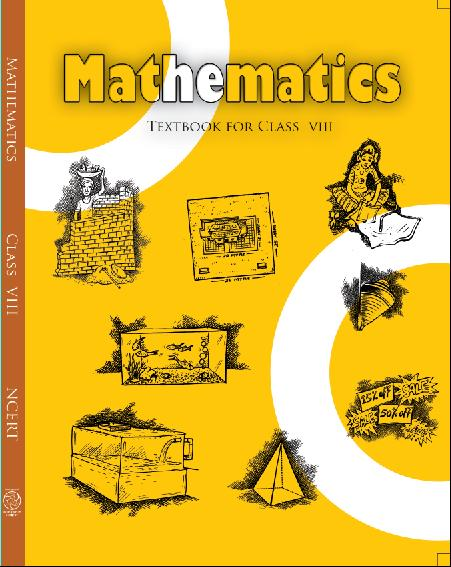## NCERT Solutions for Class 8 Maths Mensuration

###### 1.Given a cylindrical tank, in which situation will you find surface are and in which situation   volume.

(a) To find how much it can hold.

(b) Number of cement bags required to plaster it.

(c) To find the number of smaller tanks that can be filled with water from it.

Ans.  We find area when a region covered by a boundary, such as outer and inner surface area of a cylinder, a cone, a sphere and surface of wall or floor.

When the amount of space occupied by an object such as water, milk, coffee, tea, etc., then we have to find out volume of the object.

(a) Volume (b) Surface area (c) Volume

NCERT Solutions for Class 8 Maths Exercise 11.4

###### 2. Diameter of cylinder A is 7 cm and the height is 14 cm. Diameter of cylinder B is 14 cm and height is 7 cm. Without doing any calculations can you suggest whose volume is greater? Verify it by finding the volume of both the cylinders. Check whether the cylinder with greater volume also has greater surface area.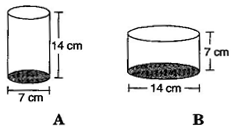Ans. Yes, we can say that volume of cylinder B is greater, since radius of cylinder B is greater than that of cylinder A (and square of radius gives more value than previous).

Diameter of cylinder A = 7 cm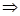Radius of cylinder A =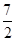cm

And Height of cylinder A = 14 cm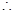Volume of cylinder A =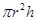=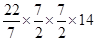= 539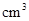Now Diameter of cylinder B = 14 cmRadius of cylinder B =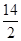= 7 cm

And Height of cylinder B = 7 cmVolume of cylinder A ==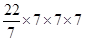= 1078Total surface area of cylinder A

=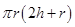[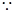It is open from top]

=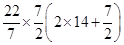=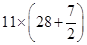=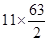= 346.5 cm2

Total surface area of cylinder B

=[It is open from top]

=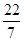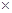7 (27 + 7)

= 22(14 + 7) = 2221 = 462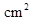Yes, cylinder with greater volume also has greater surface area.

###### 3. Find the height of a cuboid whose base area is 180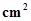and volume is 900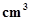?

Ans. Given: Base area of cuboid = 180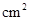and Volume of cuboid = 900We know that,

Volume of cuboid =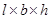900=180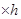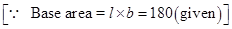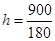= 5 m

Hence the height of cuboid is 5 m.

NCERT Solutions for Class 8 Maths Exercise 11.4

###### 4. A cuboid is of dimensions 60 cm54 cm30 cm. How many small cubes with side 6 cm can be placed in the given cuboid?

Ans. Given: Length of cuboid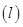= 60 cm, Breadth of cuboid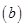= 54 cm and

Height of cuboid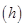= 30 cm

We know that, Volume of cuboid

== 605430And Volume of cube = (Side)3

= 666Number of small cubes

=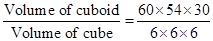= 450

Hence required cubes are 450.

NCERT Solutions for Class 8 Maths Exercise 11.4

###### 5. Find the height of the cylinder whose volume if 1.54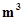and diameter of the base is 140 cm.

Ans. Given: Volume of cylinder = 1.54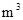and Diameter of cylinder = 140 cmRadius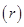=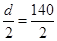= 70 cm

Volume of cylinder =1.54 =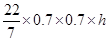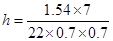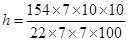= 1 m

Hence height of the cylinder is 1 m.

NCERT Solutions for Class 8 Maths Exercise 11.4

###### 6. A milk tank is in the form of cylinder whose radius is 1.5 m and length is 7 m. Find the quantity of milk in liters that can be stored in the tank.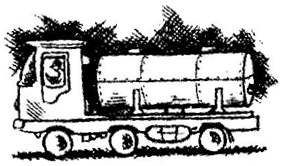Ans. Given: Radius of cylindrical tank= 1.5 m and Height of cylindrical tank= 7 m

Volume of cylindrical tank ==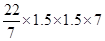= 49.5= 49.51000 liters

[1= 1000 liters]

= 49500 liters

Hence required quantity of milk is 49500 liters.

NCERT Solutions for Class 8 Maths Exercise 11.4

###### 7. If each edge of a cube is doubled,

(i) how many times will its surface area increase?

(ii) how many times will its volume increase?

Ans. (i) Let the edge of cube be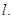Since, Surface area of the cube (A) =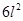When edge of cube is doubled, then

Surface area of the cube (A’)

=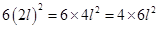A’ = 4A

Hence surface area will increase four times.

(ii) Volume of cube (V) =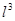When edge of cube is doubled, then

Volume of cube (V’)=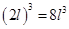V’ = 8V

Hence volume will increase 8 times.

NCERT Solutions for Class 8 Maths Exercise 11.4

###### 8. Water is pouring into a cuboidal reservoir at the rate of 60 liters per minute. If the volume of reservoir is 108, find the number of hours it will take to fill the reservoir.

Ans. Given: volume of reservoir = 108Rate of pouring water into cuboidal reservoir = 60 liters/minute

=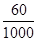/minute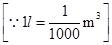=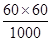/hourwater filled in reservoir will take = 1 hour1water filled in reservoir will take =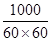hours108water filled in reservoir will take =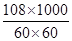hours = 30 hours

It will take 30 hours to fill the reservoir.

## NCERT Solutions for Class 8 Maths Exercise 11.4

NCERT Solutions Class 8 Mathematics PDF (Download) Free from myCBSEguide app and myCBSEguide website. Ncert solution class 8 Mathematics includes text book solutions from Class 8 Maths Book . NCERT Solutions for CBSE Class 8 Maths have total 16 chapters. 8 Maths NCERT Solutions in PDF for free Download on our website. Ncert class 8 solutions PDF and Maths ncert class 8 PDF solutions with latest modifications and as per the latest CBSE syllabus are only available in myCBSEguide.

## CBSE app for Class 8

To download NCERT Solutions for class 8 Social Science, Computer Science, Home Science,Hindi ,English, Maths Science do check myCBSEguide app or website. myCBSEguide provides sample papers with solution, test papers for chapter-wise practice, NCERT solutions, NCERT Exemplar solutions, quick revision notes for ready reference, CBSE guess papers and CBSE important question papers. Sample Paper all are made available through the best app for CBSE students and myCBSEguide website.Test Generator

Create question paper PDF and online tests with your own name & logo in minutes.myCBSEguide

Question Bank, Mock Tests, Exam Papers, NCERT Solutions, Sample Papers, Notes

### 10 thoughts on “NCERT Solutions for Class 8 Maths Exercise 11.4”

1. helping but also confusing

2. Hi this is very

3. Gud job

4. Thanks for this great help.

5. thank you soo much

6. thank you so much for this ncert solutions

7. Cannot understand even a single thing ???

8. This app is super